This video was brought to you by GeneralPAC.com, making power systems Intuitive, Open and Free for Everyone, Everywhere. Consider subscribing and supporting through patreon.com/GeneralPAC. This is a mechanism for you to support us financially so we can continue making high quality power system video tutorials. Our corporate sponsor for this topic is AllumiaX.com from Seattle, Washington. Contact them for industrial and commercial power system studies.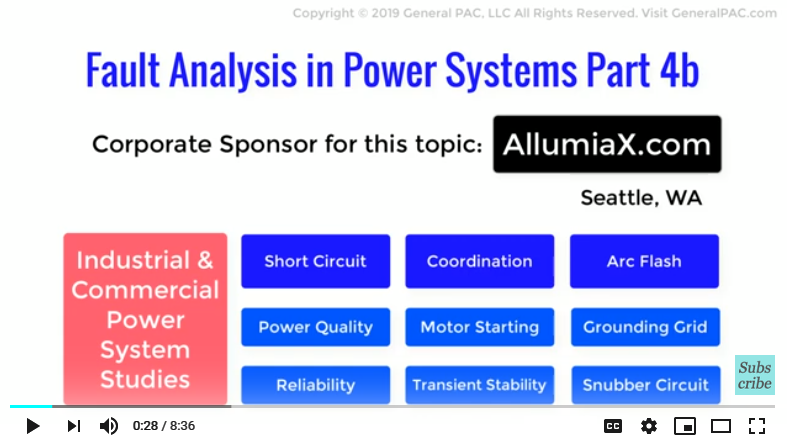In this video we will see how we can actually take a 3LG fault quantities which is fault current and voltage quantities which occur on the LV side of the transformer but we’ll reflect the same fault on the HV side.

So first let’s have a recap of Part 3a in which we calculated fault current and voltage quantities for a three line to ground fault on the 13.8 kV bus.

For the 3LG fault type, we found that:

Ia(1) = -j6.67 pu And

Ia(2) = Ia(0) = 0

To clarify, this was on the LV side of the transformer. And remember that there are no negative and zero sequence components in a 3LG fault because it’s a perfectly balanced fault type.

That can be expressed as 6.67 angle -90 in polar form. It’s also important to note the subscript – the lower case indicates fault current on LV side. The upper case will represent fault current on the HV Side.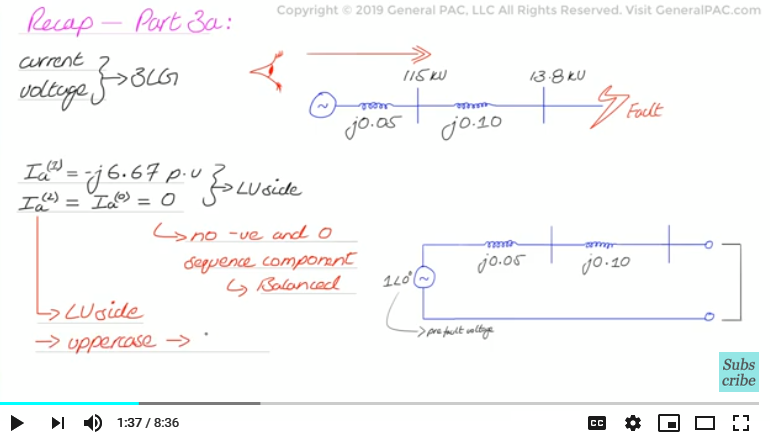We will now proceed by reflecting these per unit current quantities on the high voltage side and then we will multiply them with the appropriate base value of current too find the actual ampere current value.

So, given that we have a Dy1 or a DAB transformer connection, to determine the HV positive sequence components, we will shift the positive sequence component by +30 degrees and that can be done by multiplying a LV fault current with a value of 1 angle + 30 degrees. 6.67 per unit at the angle of -60 degrees is the positive sequence current on the high voltage side of the transformer.

For the zero sequence component and the negative sequence component however, It will be nonexistent because three line to ground fault again is a balanced symmetrical fault and there are no negative and zero sequence components in symmetrical faults. Now to convert these per unit values to actual ampere values in sequence components we have to set up a base value, but this time because we are working on the HV side, so we divide the power value of 30 MVA, by the HV voltage side of the transformer, 115 kV and we get 150.6 Amps

And we just multiply out per unit quantities with the base value to get the ampere quantities for the sequence components.

We get 1004 angle -60 amperes for positive sequence current.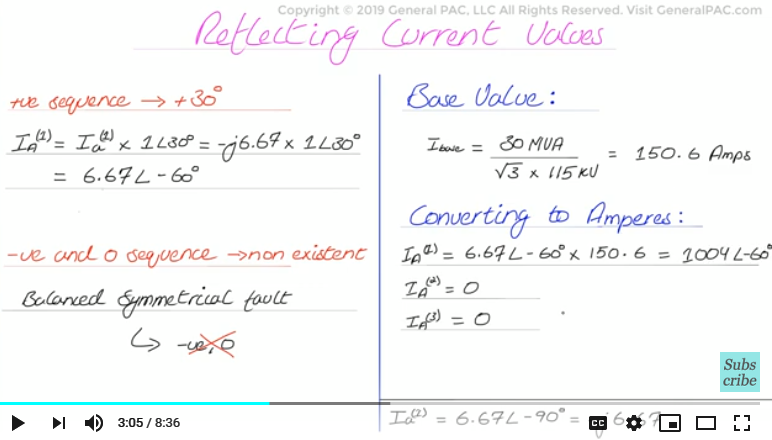And then back to our familiar transformation equations, these quantities can be transformed to phase current quantities but please note that we are removing the zero and negative sequence currents from these transformation equations because they do not exist on the high voltage side, and we get the following.

Plugging in the values of a operator and a squared operator as well as sequence component values, , we get the following. We find that the line A current, to 1004 A angle -60, IB = 1004 A angle 180 degrees and IC equal to 1004 A angle 60 degrees.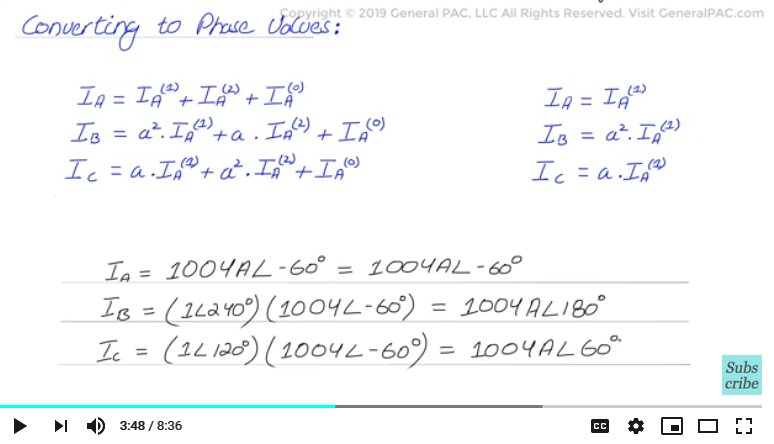This lines up very well with current flow path on the HV side. The fault current will flow on HV Line A, B and C for a LV 3LG fault involving all three lines. The reason why the fault current will flow to line A, B and C on the HV side of the transformer is because of the specific type of DY1 transformer connection as mentioned in Part 4a of this series which can also be visually seen in the diagram shown.

It also makes sense that negative and zero sequence currents do not exist on the HV side. Even if we tried to calculate the negative and zero sequence current using phase A, B, and C current quantities on the HV side, we still get zero.For example: The equation for zero sequence current is one third of Line A current + Line B current + Line C Current as shown: and if we plug in these values we simply get 0 for the zero sequence current.

And similarly if we plug in the values for negative sequence current equation we also get 0 for negative sequence current. Remember that the three line to ground fault is a perfectly balanced fault type and it will be perfectly balanced on the low voltage side of the transformer as well as on the high voltage side of the transformer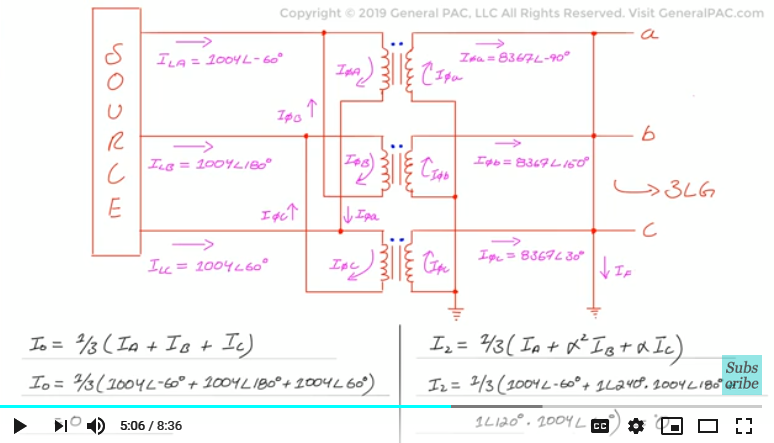Next, we will calculate the sequence voltages quantities, again reflected on the high voltage side of the transformer.

To do that, we get back to our faulted sequence network diagram for a three line to ground fault and apply KVL in the positive sequence network.

We can calculate the positive sequence voltage by considering the voltage of the positive sequence source 1 angle 0 degrees and the impedance of the source which is j.05 pu, since j0.05 is the only impedance on HV side. The positive seq. voltage for a 3LG fault is simply the source voltage minus the voltage drop across the positive sequence impedance on the high voltage side.

For the positive sequence voltage, we need to consider the reflected positive sequence current on the HV side, which is 6.67 angle -60 degrees. So our equation becomes the following:

We get a value of 0.73 angle of -13 degrees. Zero sequence voltage and negative sequence voltage will be nonexistent because originally the zero sequence current and negative sequence current were also non-existant.

We now have to set up a base value to convert per unit voltage to sequence voltage values. V base can therefore be taken as the following: Now, Multiplying the components with the base value we get the voltage values in sequence component form:

48.51 kV angle -13.2 degrees – remember these are still sequence voltage quantities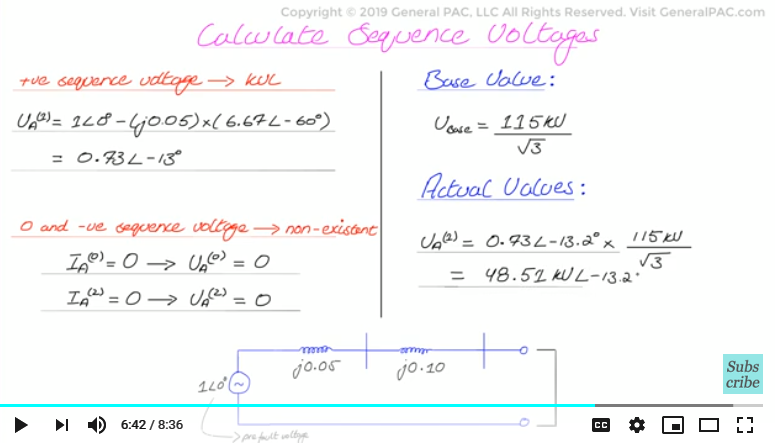We have to use, Our three legacy transformation equations to transform the sequence components voltage quantities to actual line to ground voltage quantities.And also remember that we are removing, the negative and zero sequence components from our, transformation equations:

And we plug in all the values calculated earlier, we simply get the following:

We finally get the actual line A to ground voltage being 48.51 kV at the angle of -13.2 degrees, Line B to ground voltage is 48.51 kV∠"-" 133.2° and Line C to ground voltage being 48.51 kV at the angle of 106 degrees.

Now this is a very interesting result: Since the fault was a three line to ground fault, the fault currents in each phases were equal in magnitude but displaced by 120 degrees each. This directly correlates with the voltage magnitudes as well. They are equal on each phase but also displaced by 120 degrees. And we see that there is a voltage depression on the high voltage side of the transformer despite the fault being on the low voltage side. On the high voltage side of the transformer our voltage quantities during normal operating conditions are 66 kV Line to ground but when there is a three phase fault on the low voltage side of the transformer voltage quantities are depressed and they are depressed to 48.51 kV. So they went from 66 kV to 48.51 kV so there is quite a bit of a voltage depression even on the high voltage side.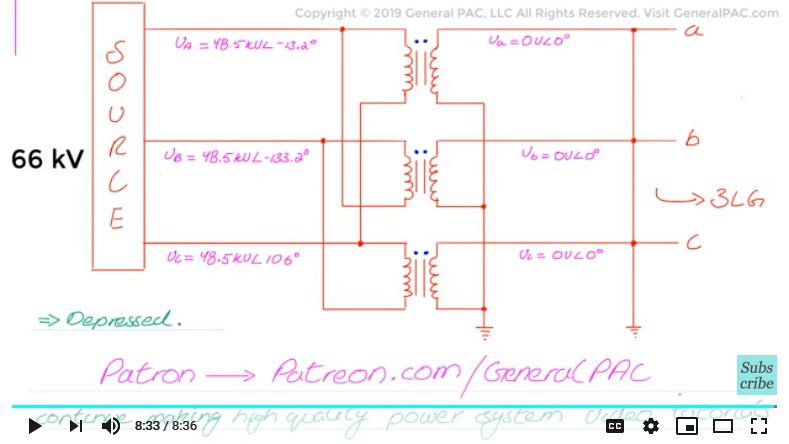We hope this video was useful and enlightening for students or professionals. Please subscribe and donate on Patreon.com/generalpac so we can continue making high quality power system video tutorials. Thank You.

Our coorporate sponsor AllumiaX,LLC published a new blog on "Fault Analysis in Power Systems". This blog covers the following topics:
• Types of Faults in Power System
• Steps to perform a Fault Analysis in a Power System
• Causes of Power System Faults#### Greetings from the GeneralPAC Team!

We make high-quality Power Systems Video Tutorials on complex topics that are free and open to everyone!  Thank you so much for supporting us through Patreon so we can continue doing good and valuable work.

What is Patreon and why do we use it?

Patreon is a fantastic portal that allows our fans and community to make monthly contribution (like Netflix subscription) so we can continue creating high-quality power systems video tutorials. In return, you get access to incredible perks like voting on future topics, getting your questions answered, access to VIP Q/A webinars with the creators of GeneralPAC, and much more! We THANK YOU for supporting us

Why do we need your support?

An incredible amount of time and effort is needed to develop high-quality video tutorials. Each video (Part 1 for example) takes approximately 10 hours to complete which includes learning the concept ourselves, brainstorming creative ways to teach and explain the concepts, writing the script, audio recording, video recording, and editing. It's no wonder why Hundreds-of-Thousands of people have watched, liked, subscribed, and left positive comments on Youtube channel. Your support truly makes all the difference.